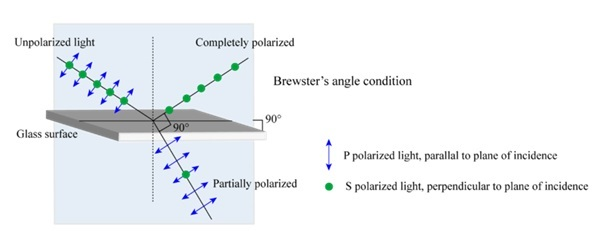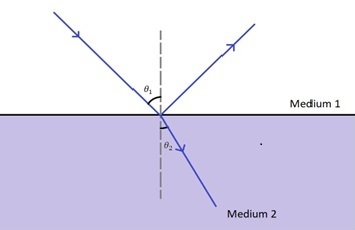# Brewster's Law: Definition, Equation, Examples

## Introduction

Brewster’s law is studied in optics. the science of light. If light waves could pass through all materials perfectly, you wouldn’t be able to see anything. All materials would turn invisible. On the other hand, if materials reflected all light perfectly, they would always appear white instead of coloured. Colours are visible only because materials absorb particular wavelengths and reflect others. In practicality, all materials reflect, transmit, or absorb lights selectively.

At the same time, light waves with a particular characteristic can be transmitted completely through a particular surface. This involves the incidence of light of a particular polarisation to be incident on a surface at a certain angle then it be transmitted completely. First, let us understand what polarisation is.

If you recall, light is an electromagnetic wave and thus, comprises electric and magnetic fields. The direction in which the electric field component of light oscillates defines its direction of polarisation, or simply, its polarisation. Now, we can discuss how perfect transmission of light can take place.

## What is Brewster’s Law?

When light is incident on the boundary of transparent surfaces, the amount of light reflected and transmitted depends on the refractive indices of the medium and the polarization of the incident waves. Brewster’s law is a relation that provides us with the angle of incidence at which total transmission will occur.

Example: A glass has a refractive index of 1.5, if placed in the air, Brewster’s angle will come out to be approximately 56°. Interestingly, note that since Brewster’s angle depends on the refractive indices of both the mediums, its value for air-to-glass will be different from, say, water-to-glass.

Another important point to note is that when unpolarized light is incident at the Brewster angle, then the reflected component is perfectly polarised in one particular direction, while the refracted ray is partially polarised. This allows us to produce light waves polarised in a particular direction via reflection.Sobarwiki, Brewster's angle polarization, marked as public domain, more details on Wikimedia Commons

## Brewster Law Formula

If we represent the refractive indices of the first and second media by $\mathrm{n_1}$ and $\mathrm{n_2}$, respectively, then, Brewster’s angle is given by −

$$\mathrm{\theta_B=\frac{n_2}{n_1}}$$

Recall Cauchy’s relation. The refractive index of a material is not a universal constant. Instead, it is itself a function of wavelength. Thus, Brewster’s angle is different for lights of different wavelengths. However, the change in Brewster’s angle is quite small for the visible region of light.

## Brewster Angle Derivation

An elegant and simple derivation of Brewster’s angle can be arrived at using Snell’s law.

When light from a material of refractive index $\mathrm{n_1}$ is incident on a material with refractive index $\mathrm{n_2}$ at an angle $\mathrm{\theta_1}$, then the angle of refraction $\mathrm{\theta_2}$ is related to these quantities as −

$$\mathrm{n_1\:sin\:\theta_1 =n_2\:sin \: \theta_2}$$Now, as it turns out, how the light waves physically interact with the medium, zero reflection occurs when $\mathrm{\theta_2=90^{\circ}-\theta_1}$. Thus,

$$\mathrm{n_1\:sin\:\theta_1 =n_2\:sin \: (90^{\circ}-\theta_1)}$$

$$\mathrm{\frac{sin\:\theta_1}{cos\: \theta_1}=\frac{n_2}{n_1}}$$

Now, sine over cosine is nothing but the tangent. And $\mathrm{θ_1}$ here is just the Brewster angle $\mathrm{θ_B}$. Therefore,

$$\mathrm{tan\:\theta_B=\frac{n_2}{n_1}}$$

Or,

$$\mathrm{\theta_B=\frac{n_2}{n_1}}$$

A more comprehensive proof of this relation can be arrived at using Maxwell’s equations, the associated boundary conditions, and Fresnel relations.

## Relation Between Brewster Angle and Critical Angle

Another concept you must have come across is that of the critical angle. The critical angle for the interface of two media is defined as the angle at which, the refracted ray lies at an angle of $\mathrm{90^{\circ}}$ with the normal. That is, beyond the critical angle, all light is reflected, and no refraction takes place. This is known as total internal reflection.

Critical angle can be found by a simple application of Snell’s law. If $\mathrm{\theta_C}$ is the critical angle, then,

$$\mathrm{n_1\:sin\:\theta_C =n_2\:sin\: 90^{\circ}=n_2}$$

Therefore,

$$\mathrm{sin\:\theta_C=\frac{n_2}{n_1}}$$

We can equate the fraction on the right-hand side to the tangent of Brewster’s angle. Thus,

$$\mathrm{sin\:\theta_C=tan\:\theta_B}$$

This is the relation between Brewster angle and the critical angle.

## Application of Brewster’s Law

Brewster’s law finds application in a wide variety of scenarios −

• You must have heard of polarised sunglasses. These sunglasses are coated with films of specific refractive indices such that light reflected from surfaces gets blocked out via reflection. This reduces glare.

• Photographers place polarizing filters in front of their lenses, which reduces glare from horizontal surfaces like water and allows them to photograph objects beneath a reflecting surface. For instance, if you have come across images of lakes that show the fishes and the fauna inside the lake clearly, it was likely captured via a polarizing lens.

• Windows that only allow you to see from one side while being reflective on the other side are based on the concept of polarisation.

• Holograms are recorded by the interference of light waves. If unwanted reflection were to occur, then there would be noise. Instead, the LASER beam used to record the Hologram is polarised and it incident at the Brewster angle. This makes the resulting hologram clearer.

• While studying optics of different materials, light incident on the Brewster angle will be transmitted perfectly, reducing unwanted reflective loss.

## Conclusion

We know that no material is a perfect reflector, absorber, or transmitter of light. If there were such materials, they would be either invisible, totally white, or black. Instead, portions of light are always reflected or absorbed. The light of a particular polarization can be transmitted perfectly. Note that the polarization of a light wave is the direction in which its electric field component oscillates. When light polarized in a particular direction is incident on the boundary of transparent interface at a certain angle, no reflection is absorbed. This angle is known as the Brewster angle, and the relation between this angle and the refractive indices of the two materials involved is given by Brewster’s law. It is given by

$$\mathrm{\theta_B=\frac{n_2}{n_1}}$$

Since the refractive index depends on the wavelength, Brewster’s angle differs for lights of different wavelengths incident on the same interface. Further, Brewster’s angle can be connected to the critical angle, which is the angle at which total internal reflection occurs.

$$\mathrm{sin\:\theta_C =tan\:\theta_B}$$

Brewster’s law is used in a plethora of situations. Polarising sunglasses, polarising filters, one-way windows, and hologram recording are some of the many examples where Brewster’s law comes in handy.

## FAQs

Q1. How is the refractive index connected to wavelength?

Ans. Cauchy’s equation gives the relation of refractive index with wavelength.

$$\mathrm{n=A+\frac{B}{\lambda^2}+\frac{C}{\lambda^4}+.....}$$

Here, A,B, and C are constants, while λ is the wavelength of the light.

Q2. Which polarization direction is reflected when unpolarised light falls at Brewster’s angle on an interface?

Ans. The light waves which are polarised perpendicular to the plane of incidence will be reflected. This is also known as s-polarisation.

Q3. What is meant by unpolarized light?

Ans. Unpolarized light is made up of light waves polarised in all possible directions. Thus, no polarization is equivalent to $\mathrm{360^{\circ}}$ polarization.

Q4. How do we achieve polarised light?

Ans. Various materials only allow the light of one particular polarization to pass through. If we want to achieve light polarized in one particular direction, we should place such materials in the path of the light beam. There are various other methods to achieve polarized light as well. We can produce polarized light via reflection, scattering, or even refraction.

Q5. Does polarization affect the intensity of the light wave?

Ans. Yes. the intensity of light passing through a polarizer is related to the intensity of the incident beam θ . The intensity of unpolarized light is halved when it passes through a polarizer.

Updated on: 18-Apr-2023

211 Views